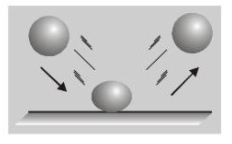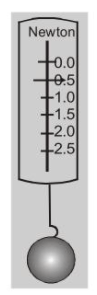Download the eSaral app and start learning from Kota's top IITians and doctors.

# FORCE

A force is a push or a pull.  since push or a pull has both magnitude and direction, so that force is a vector quantity.  Forces occur in pairs. If object A exerts a force on object $\mathrm{B},$ then $\mathrm{B}$ also exerts a force on $\mathrm{A}$. For example, when a bat strikes a ball the bat exerts a force on the ball, but the ball exerts a force on the bat also.  A force can cause an object to accelerate. If you kick a foot ball the ball's velocity changes while your foot is in contact with it.  A force can deform an object. As you can see from fig. The ball when hits the floor, is deformed by the contact force exerted on it by the floor. The floor is deformed too, but since it is harder than the ball, its deformation is not as noticeable.Property $4,$ that a force causes an object to be deformed is often used to measure a force. This is the principle of a spring scale. A spring scale indicates the amount of the spring is stretched or compressed. The magnitude of this force is proportional to the amount of the spring is stretched (or compressed), and the direction of the force is along the spring. The scale may be calibrated to read in newton (N), units of force in SI system is newton and in $\mathrm{C} . \mathrm{G} . \mathrm{S} .$ system is dyne. 1 newton $=10^{5}$ dyne. $1 \mathrm{kg} \mathrm{wt}=\mathrm{g}$ newton. Dimensional formula of force is $\mathrm{M}$ L $\mathrm{T}^{-2}$ Dimensional formula of force is $\mathrm{M} \mathrm{L} \mathrm{T}^{-2}$## TYPES OF FORCES

(a) Field Forces or Range Forces: These are the forces in which contact between two objects is not necessary. Ex. (i) Gravitational force between two bodies. (ii) Electrostatic force between two charges. (iii) Weight of a body. (b) Contact Forces: When a force involves direct contact between two bodies. One body exerts a force on another because of the contact between them. These forces originate with the atoms of each body - each atom exerts a force on its neighbour (which may be an atom of another body). A contact force can be maintained only if it does not exceed the interatomic forces within either of the bodies; otherwise the binding between atoms can be overcome and the string or the surface will split into pieces. Contact forces include the pushes or pulles with your hand. Ex. (i) Force of rope pulling on a block to which it is tied. (ii) Tension in string, and normal forces. (iii) Frictional force (c) Attachment to Another Body: Tension (T) in a string and spring force (F=kx) comes in this group.